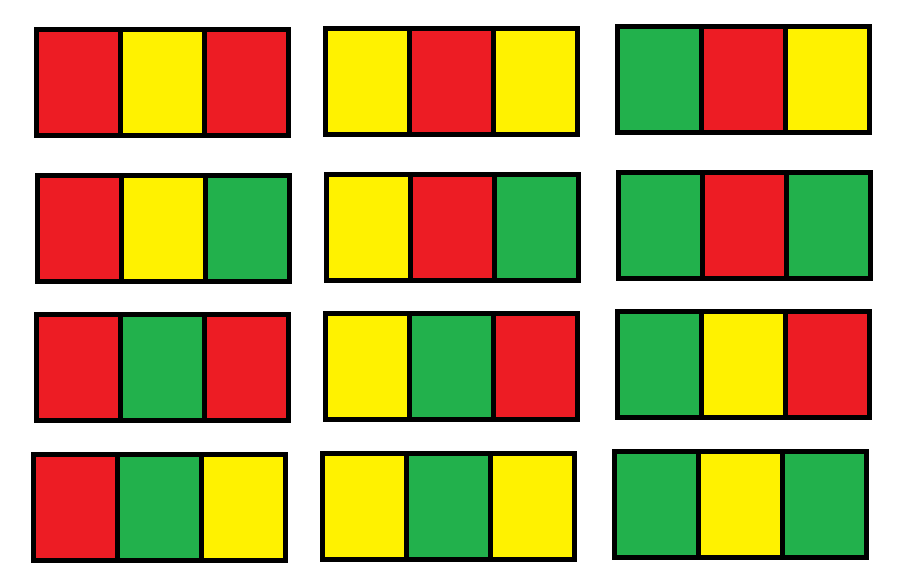# 给 N x 3 网格图涂色的方案数

## 1411. 给 N x 3 网格图涂色的方案数 (Hard)

```输入：n = 1```

```输入：n = 2

```

```输入：n = 3

```

```输入：n = 7

```

```输入：n = 5000

```

• `n == grid.length`
• `grid[i].length == 3`
• `1 <= n <= 5000`

[动态规划]